# AP Chemistry : Equilibrium and Thermodynamics

## Example Questions

### Example Question #1 : Equilibrium And Thermodynamics

Consider the following gasesous, reversible, exothermic reaction: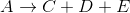What could be done to increase the equilibrium concentration of species C?

Increase the reaction temperature

Increase the amount of species E

Increase the amount of species A

Decrease the amount of species A

Increase the amount of species A

Explanation:

According to Le Chatelier's principle, any changes in concentration or pressure to a system at equilibrium will cause the system to readjust to a new equilbrium. The addition of a reactant will cause the reaction to shift to the right, increasing the equilibirum concentration of the products. Thus, adding additional reactant A would increase the equlibrium concentration of product C.

Removing species A or adding species E will drive the reaction to the left, reducing the amount of species C. Since the reaction is exothermic, heat can be considered a product. Thus, increasing the temperature will also shift the reaction to the left.

### Example Question #1 : Chemical Equilibrium

Consider the following chemical reaction.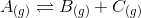This reaction is allowed to equilibrate at some volume and pressure.

Which of the following actions will not shift the equilibrium toward the products?

AddingIncreasing the volume

RemovingDecreasing the pressure

Explanation:

Adding an inert gas will not shift the equilibrium toward the products, but neither will it shift the equilibrium toward the reactants. It has no effect because the partial pressure of each species,,, and, will still remain constant. Since the partial pressures are constant, we know from the ideal gas law that the concentration of each species remains constant. For example, for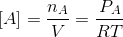### Example Question #41 : Reactions And Equilibrium

If formate is added to a solution of formic acid in water, which of the following changes would occur?

Decreased pH

Decreased pKa

Increased pKa

Increased pH

There will be no change

Increased pH

Explanation:

Because formic acid is a weak acid, it will exist in equilibrium with its conjugate base, formate, while in water according to the following reaction: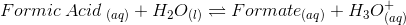If formate is added to this solution, then according to Le Chatlier's principle, the reaction will shift towards the left. Thus, the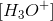in solution will decrease and the pH will consequently increase. Furthermore, the addition of formate has no effect on the pKa.

### Example Question #1 : Chemical Equilibrium

For a given chemical reaction,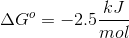. What is the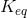for this reaction at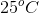?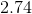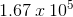There is not enough informationExplanation:

To answer this question, it is necessary to use following equation: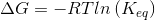We can solve for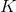by rearranging the equation to the following: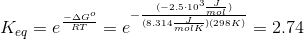### Example Question #1 : Chemical Equilibrium

A reaction at equilibrium releases 25 Joules of heat energy at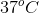. What is the entropy change for this same reaction?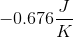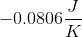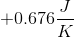There is not enough information to answer the questionExplanation:

To solve this problem, we must make use of the following equation: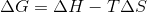At equilibrium, the value of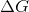is 0. Therefore, we can simplify the equation.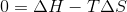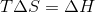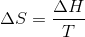We must also convert temperature from degress Celsius into Kelvin.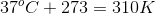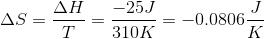### Example Question #1 : Chemical Equilibrium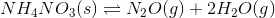Which of the following is the correct equilibrium expression for the given reaction?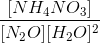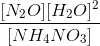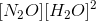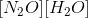Explanation:

For this question, we're asked to determine the correct equilibrium expression for a given reaction that is in equilibrium.

To begin with, we need to remember that pure solids and liquids are omitted from the equilibrium expression. Only gases and aqueous components of a reaction are included. The reason for this is because in a reaction at equilibrium, a pure solid or liquid will not have any appreciable change in concentration. Because their concentrations remain essentially unchanged, their value gets incorporated into the constant.

Also remember that an equilibrium expression is represented by the products divided by the reactants, each of which is raised to its stoichiometric coefficient.

In the case of the reaction given, we can see that it is already balanced. This means we are ready to set up the expression.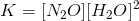Here, the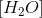term is being squared because it has a stoichiometric coefficient ofin the equilibrium reaction. Also notice that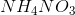does not show up in the expression because it is a solid.

### Example Question #1 : Equilibrium And Thermodynamics

The reaction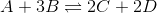is at equilibrium. You have measured the concentration ofandto be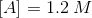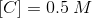What is the equilibrium constant,?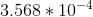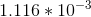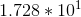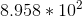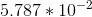Explanation:

Recall that the rate constant for the equilibrium reaction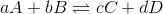is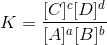, always remembering that it is products over reactants.

Since we are at equilibrium, for every mol ofwe have, we have 3 mols of. Therefore,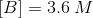Likewise, for every 2 mols ofwe have, we have 2 mols of. Therefore,Plugging all of this into the above equation we get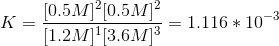### Example Question #42 : Reactions And Equilibrium

1.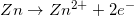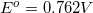2.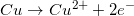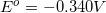3.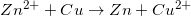What is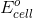? Is reaction 3 spontaneous?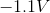, spontaneous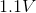, spontaneous, nonspontaneous, nonspontaneous, nonspontaneous

Explanation: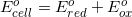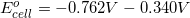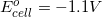There are two concepts to consider in this problem.  First, the question asks for the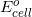.  Reaction 3 has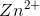being reduced so the potential for the half-reaction becomes negative.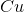half-reaction appears the same in reaction 3 so the potential is the same. Second, negative voltages indicate non-spontaneity and positive voltages are spontaneous.

### All AP Chemistry Resources